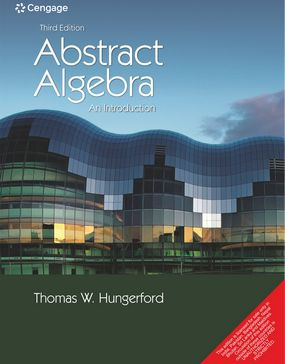###### Home
>

Higher Education### Abstract Algebra: An Introduction, 3E (Exclusive for Shri Adhya)

Author(s): Thomas W. Hungerford

ISBN: 9788131525722

Edition: 3rd

#### Rs. 995

Binding: Paperback

Pages: 620

Trim Size : 241 x 181 mm

Abstract Algebra: An Introduction is set apart by its thematic development and organization. The chapters are organized around two themes: arithmetic and congruence. Each theme is developed first for the integers, then for polynomials, and finally for rings and groups. This enables students to see where many abstract concepts come from, why they are important, and how they relate to one another. New to this edition is a "groups first" option that enables those who prefer to cover groups before rings to do so easily.

• Thematic Development The Core Course (Chapters 1–8) is organized around two themes: Arithmetic and Congruence
• Congruence The Congruence theme is strongly emphasized hi the development of
• quotient rings and quotient groups. Consequently, students can see more clearly that
• ideals, normal subgroups, quotient rings, and quotient groups are simply an extension
• of familiar concepts in the integers, rather than an unmotivated mystery.
• Coverage The breadth of coverage in this edition is substantially the same as in
• the preceding ones, with one minor exception.
• Useful Appendices These contain prerequisite material (e.g., logic, proof, sets,
• functions, and induction) and optional material that some instructors may wish to
• introduce (e.g., equivalence relations and the Binomial Theorem).
• Endpapers The endpapers now provide a useful catalog of symbols and notations

Part 1 T he Core Course

1. Arithmetic in Z Revisited.

2. Congruence in Z and Modular Arithmetic

3. Rings

4. Arithmetic in F [x]

5. Congruence in F [x] and Congruence-Class Arithmetic

6. Ideals and Quotient Rings

7. Groups

8. Normal Subgroups and Quotient Groups

9. Topics in Group Theory

10. Arithmetic in Integral Domains

11. Field Extensions

12. Galois Theory

Part 3 Excursions and Applications 8. Experiments.

13. Public-Key Cryptography

14. The Chinese Remainder Theorem

15. Geometric Constructions

16. Algebraic Coding Theory

Part 4 Appendices

A. Logic and Proof

B. Sets and Functions

C. Well Ordering and Induction

D . Equivalence Relations

E . The Binomial Theorem

F . Matrix Algebra

G . Polynomials

Thomas W. Hungerford

Thomas W. Hungerford received his M.S. and Ph.D. from the University of Chicago. He has taught at the University of Washington and at Cleveland State University, and is now at St. Louis University. His research fields are algebra and mathematics education. He is the author of many notable books for undergraduate and graduate level courses.